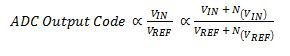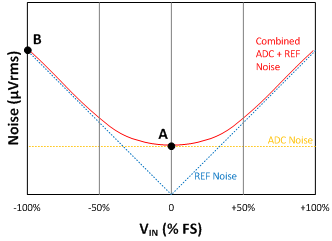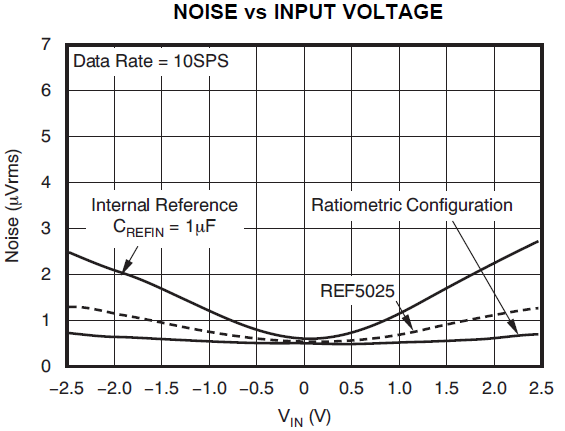Other Parts Discussed in Post: ADS1259

Have you ever evaluated the noise performance of an ADC and found that your measured performance differs from the specified performance given in the device datasheet? Achieving high resolution in a precision data-acquisition system requires some understanding of analog-to-digital converter (ADC) noise. It is important to know how the datasheet specifies noise performance and how external noise sources may affect overall system performance. One example of such a noise source is the power-supply noise my colleague Ryan Andrews discussed in his post, “Caution! Your ADC may be only as good as its power supply.” In this post, I’ll look at how reference noise affects DC noise performance in delta-sigma ADCs.

You can specify and measure the DC noise performance of an ADC with the positive and negative inputs shorted to a mid-supply voltage, as shown in Figure 1. By measuring noise under this condition, the noise in the ADC’s output codes is nearly immune to changes in reference voltage, reference noise or input-signal noise. While this test condition is more of an ideal scenario than a practical application, it does provide a good representation of ADC noise performance isolated from some external noise sources.Figure 1: ADC noise performance test (and debugging) configuration

Tip: When debugging, begin evaluating the system’s noise performance with a shorted-input test to assess the isolated ADC noise performance before moving on to other system noise performance tests.

How reference noise affects ADC DC noise performance

This effect is related to the basic task of an ADC, which is to provide an output code that is a representation of the ratio of the input-signal voltage to the reference voltage. Both input and reference voltages add a noise term to this ratio, as shown in Equation 1:(1)

The effect of input signal noise,, on ADC conversion results is fairly straightforward. The ADC will capture any noise not filtered out – either with an external resistor-capacitor (RC) filter or with the delta-sigma ADC’s digital filter. You can observe this in the output codes, sincehas a direct effect on the ratio in Equation 1.

Tip: Make sure that the input signal is a low-noise source when evaluating ADC noise performance, since the input signal’s noise directly affects the ADC’s output result.

The effect of reference noise,, on the ADC conversion result is not quite as straightforward, however, becauseappears in the denominator. When the numerator is equal to zero (as is the case when the ADC inputs are shorted), the ratio will always equal zero and theterm will not effect on the ratio. When the numerator is nearly equal to the denominator,will have a strong effect on the ratio. When ratio values are between 0 and 1, the effect ofis weighted by the value of the ratio. Figure 2 shows the resulting characteristic behavior.Figure 2: ADC and reference noise vs. input voltage

When adding reference noise to the ADC’s noise contribution using root-sum-of-squares addition, the combined noise performance is a function of the input voltage, which increases for larger positive or negative input voltages. The important points to notice on the curve in Figure 2 are:

• Point A, which is the ADC noise measured with shorted inputs given in the ADC’s datasheet.
• Point B, which is the total bandwidth-limited reference noise, typically limited by the ADC’s digital filter bandwidth.

You can calculate the reference noise (point B) if you know the noise-spectral density and noise bandwidth for the reference source; otherwise, applying a full-scale voltage input to the ADC and measuring the noise performance will generally be a good measure of reference noise.

How to select a reference voltage source

A low-noise reference is important for achieving low-noise/high-resolution performance across the full ADC input range. The reference-noise requirements will depend on the system’s targeted resolution, the input-signal span and the data rate (as this usually limits both the input and reference-noise bandwidth). The system can tolerate additional reference noise when the noise bandwidth is limited by a slower data rate, or if the input-signal span is limited to a small percentage of the ADC’s full-scale range.

Many delta-sigma ADCs include an integrated reference that provides sufficient performance for most applications. For more demanding applications, using an external reference may improve noise performance for inputs near the positive and negative full-scale range. External precision references can achieve lower noise performance because of their higher power consumption. Figure 3 compares the 24-bit ADS1259 delta-sigma ADC’s noise performance with the internal reference source, an external REF5025 voltage source, and a ratiometric reference source.Figure 3: ADS1259 noise performance with internal, external and ratiometric reference sources

While external references may achieve better noise performance than their integrated counterparts, ratiometric reference configurations can perform even better. A ratiometric configuration shares the same voltage source for the reference voltage and input-signal excitation. By sharing a common voltage and noise source,andfrom Equation 1 tend to cancel each other out in the ratio.

Next time you’re evaluating an ADC’s noise performance, make sure to consider the reference noise effects. Also, whenever a sensor requires an excitation source, always consider a ratiometric measurement implementation.